# Samacheer Kalvi Books: Tamilnadu State Board Text Books Solutions

## Samacheer Kalvi 9th Maths Chapter 7 Mensuration Notes PDF Download: Tamil Nadu STD 9th Maths Chapter 7 Mensuration NotesSamacheer Kalvi 9th Maths Chapter 7 Mensuration Notes PDF Download: Tamil Nadu STD 9th Maths Chapter 7 Mensuration Notes

We bring to you specially curated Samacheer Kalvi 9th Maths Chapter 7 Mensuration Notes PDF which have been prepared by our subject experts after carefully following the trend of the exam in the last few years. The notes will not only serve for revision purposes, but also will have several cuts and easy methods to go about a difficult problem.

 Board Tamilnadu Board Study Material Notes Class Samacheer Kalvi 9th Maths Subject 9th Maths Chapter Chapter 7 Mensuration Format PDF Provider Samacheer Kalvi Books

## How to Download Samacheer Kalvi 9th Maths Chapter 7 Mensuration Notes PDFs?

2. Click on the Samacheer Kalvi 9th Maths Notes PDF.
3. Look for your preferred subject.
4. Now download the Samacheer Kalvi 9th Maths Chapter 7 Mensuration notes PDF.

## Tamilnadu Samacheer Kalvi 9th Maths Solutions Chapter 7 Mensuration Additional Questions

Exercise 7.1

Question 1.
Using Heron’s formula, find the area of a triangle whose sides are 41 m, 15 m, 25 m.
Solution: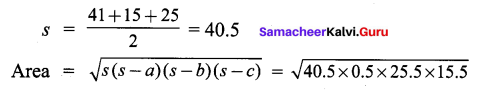Question 2.
Find the area of an equilateral triangle whose perimeter is 150 m.
Solution:
3a = 150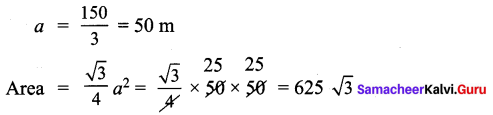Question 3.
Find the area of a quadrilateral whose sides are PQ = 15 cm, QR = 8 cm, RS = 25 cm, PS = 12 cm and LQ = 90°
Solution: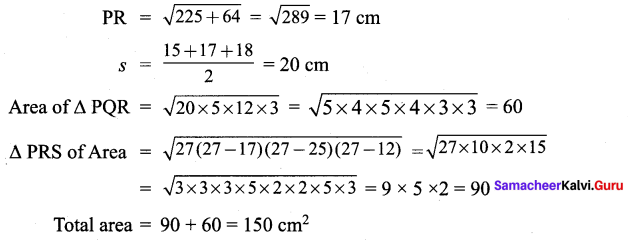Exercise 7.2

Question 1.
Find the TSA and LSA of a cuboid whose length, breadth and height are 10 cm, 12 cm and 14 cm respectively.
Solution:
TSA = 2 (lb + bh + Ih)
= 2 (10 × 12 + 12 × 14 + 10 × 14) = 2 (120 + 168 + 140) = 856 cm2
LSA = 2 (bh + Ih) = 2 (12 × 14 + 10 × 14) = 2 (168 + 140) =2 (308) = 616 cm2

Question 2.
A cuboid has total surface area of 40 m2 and its lateral surface area is 26 m2. Find the area of its base.
Solution: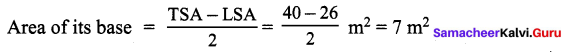Question 3.
Find the surface area of a cube whose edge is
(i) 27 cm
(ii) 3 cm
(iii) 6 cm
(iv) 2.1 cm
Solution:
(i) TSA = 6a2 = 6 × 272 = 6 × 729 = 4374 cm2
(ii) TSA = 6a2 = 6 × 32 = 54 cm2
(iii) TSA = 6a2 = 6 × 62 = 6 × 36 = 216 cm2
(iv) TSA = 6a2 = 6 × 2.12 = 6 × 4.41 = 26.46 cm2

Exercise 7.3

Question 1.
Find the volume of a cube whose surface area is a 96 cm2.
Solution:
6a2 = 96 cm2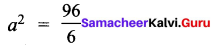a = 6
Volume = a3 = 63 = 36 × 6 = 216 cm3

Question 2.
The volume of a cuboid is 440 cm3 and the area of its base is 88 cm2, find its height.
Solution:
l × b × h = 440 cm3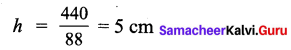Question 3.
How many 3 metre cubes can be cut from a cuboid measuring 18 m × 12 m × 9 m?
Solution: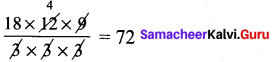Question 4.
The outer dimensions of a closed wooden box are 10 cm by 8 cm by 7 cm. Thickness
of the wood is 1 cm3. Find the total cost of wood required to make box if 1 cm3 of wood costs ₹ 2.00?
Solution:
Volume of wood = 12 × 10 × 9 – 10 × 8 × 7 = 1080 – 560 = 520 cm3 × 2.00 = ₹ 1040

## How to Prepare using Samacheer Kalvi 9th Maths Chapter 7 Mensuration Notes PDF?

Students must prepare for the upcoming exams from Samacheer Kalvi 9th Maths Chapter 7 Mensuration Notes PDF by following certain essential steps which are provided below.

• Use Samacheer Kalvi 9th Maths Chapter 7 Mensuration notes by paying attention to facts and ideas.
• Pay attention to the important topics
• Refer TN Board books as well as the books recommended.
• Correctly follow the notes to reduce the number of questions being answered in the exam incorrectly
• Highlight and explain the concepts in details.

## Frequently Asked Questions on Samacheer Kalvi 9th Maths Chapter 7 Mensuration Notes

#### How to use Samacheer Kalvi 9th Maths Chapter 7 Mensuration Notes for preparation??

Read TN Board thoroughly, make separate notes for points you forget, formulae, reactions, diagrams. Highlight important points in the book itself and make use of the space provided in the margin to jot down other important points on the same topic from different sources.

#### How to make notes for Samacheer Kalvi 9th Maths Chapter 7 Mensuration exam?

Read from hand-made notes prepared after understanding concepts, refrain from replicating from the textbook. Use highlighters for important points. Revise from these notes regularly and formulate your own tricks, shortcuts and mnemonics, mappings etc.
Share: